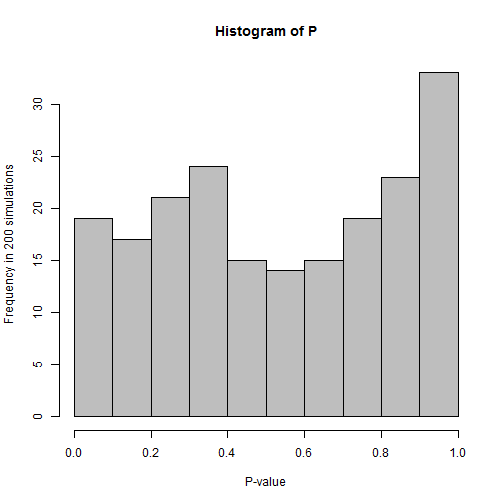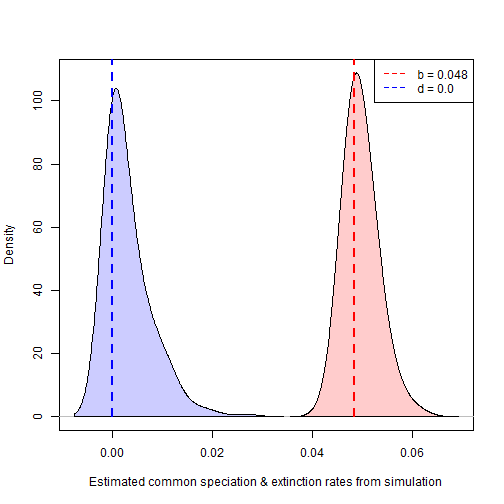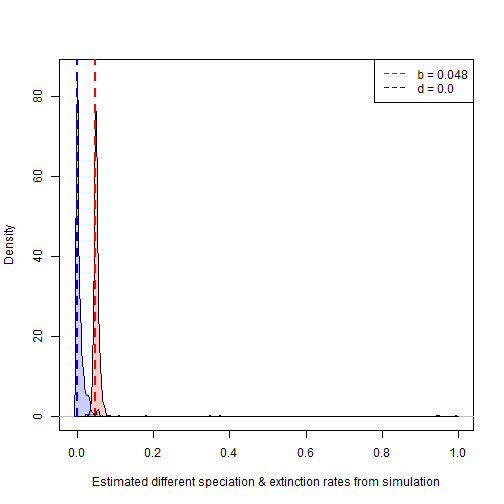## Thursday, October 26, 2017

### Comparing the speciation & extinction rates between phylogenies using `ratebytree`: Type I error & parameter estimation under the null

Earlier today I posted on comparing the rate of diversification (speciation & extinction) among phylogenetic trees.

The underlying idea is simple - we just fit one model in which each tree is permitted to have different speciation & extinction rates, followed by a second, simpler model in which all trees are constrained to have the same rate, and then we compare the likelihoods. The former model should always have a higher likelihood - but it also contains N times as many parameters, for N different trees, so we need to take this into account.

Since the simple model of all rates equal is naturally a special case of the rates-different model we can compare the two models using a likelihood-ratio test, which we expect should have a Χ2 distribution with 2N - 2 degrees of freedom.

To examine the statistical performance of the method what I'm first going to do is simulate under the null & then compute the type I error rate to its nominal rate of α = 0.05.

This is easy. In the following, I first set a birth (speciation) rate (λ) that on average should produce 250 lineages after 100 units of time. Of course, this value will vary stochastically among simulations. I'll
simulate 200 sets of three trees with a constant speciation rate & an extinction rate of μ = 0. Then, I'll fit our `ratebytree` models to each set of trees. Finally, I'll extract the P-values from the likelihood-ratio tests on each set of phylogenies. Since the null hypothesis is true, these should have a more-or-less uniform distribution and 5% or so of them should be less than or equal to 0.05.

``````library(phytools)
B<-(log(250)-log(2))/100
trees<-replicate(200,pbtree(b=B,t=100,nsim=3),simplify=FALSE)
fits<-lapply(trees,ratebytree)
``````
``````## There were 50 or more warnings (use warnings() to see the first 50)
``````
``````P<-sapply(fits,function(x) x\$P.chisq)
hist(P,col="grey",xlab="P-value",ylab="Frequency in 200 simulations")
````````````mean(P<=0.05,na.rm=TRUE)
``````
``````##  0.07
``````

We can also pull out the parameter values & see how they compare to our generating values. We might expect our density for the birth rate, λ or `b` to be centered near the generating value, and the extinction rate to be near 0.0 (although it should be slightly greater than zero as we are using bounded optimization).

``````common.bd<-t(sapply(fits,function(x) x\$common.rate.model\$rates))
``````
``````##               b           d
## [1,] 0.05156471 0.002623277
## [2,] 0.04982780 0.002574423
## [3,] 0.04919032 0.000000000
## [4,] 0.04978870 0.000000000
## [5,] 0.04923988 0.000000000
## [6,] 0.05013219 0.000000000
``````
``````b<-density(common.bd[,"b"],bw=0.0025)
d<-density(common.bd[,"d"],bw=0.0025)
plot(b,xlim=range(c(b\$x,d\$x)),ylim=range(c(b\$y,d\$y)),col="red",
xlab="Estimated common speciation & extinction rates from simulation",
ylab="Density",main="")
polygon(x=c(min(b\$x),b\$x,max(b\$x)),y=c(0,b\$y,0),col=make.transparent("red",0.2))
lines(rep(B,2),c(0,par()\$usr),col="red",lty="dashed",lwd=2)
lines(d,col="blue")
polygon(x=c(min(d\$x),d\$x,max(d\$x)),y=c(0,d\$y,0),col=make.transparent("blue",0.2))
lines(rep(0,2),c(0,par()\$usr),col="blue",lty="dashed",lwd=2)
legend(x=par()\$usr,y=par()\$usr,c(paste("b =",round(B,3)),
"d = 0.0"),col=c("red","blue"),lty=rep("dashed",2),xjust=1,yjust=1)
``````That's pretty neat. Now from the more complex, multi-rate models. Remember, these were all simulated under identical conditions so I'm just going to pool them. These densities should look fairly similar to the previous plot, although since each parameter value is estimated from a single tree, they might have more variability.

``````multi.b<-t(sapply(fits,function(x) x\$multi.rate.model\$rates[,"b"]))
multi.d<-t(sapply(fits,function(x) x\$multi.rate.model\$rates[,"d"]))
b<-density(multi.b,bw=0.0025)
d<-density(multi.d,bw=0.0025)
plot(b,xlim=range(c(b\$x,d\$x)),ylim=range(c(b\$y,d\$y)),col="red",
xlab="Estimated different speciation & extinction rates from simulation",
ylab="Density",main="")
polygon(x=c(min(b\$x),b\$x,max(b\$x)),y=c(0,b\$y,0),col=make.transparent("red",0.2))
lines(rep(B,2),c(0,par()\$usr),col="red",lty="dashed",lwd=2)
lines(d,col="blue")
polygon(x=c(min(d\$x),d\$x,max(d\$x)),y=c(0,d\$y,0),col=make.transparent("blue",0.2))
lines(rep(0,2),c(0,par()\$usr),col="blue",lty="dashed",lwd=2)
legend(x=par()\$usr,y=par()\$usr,c(paste("b =",round(B,3)),
"d = 0.0"),col=c("red","blue"),lty=rep("dashed",2),xjust=1,yjust=1)
``````The only difference, just as we predicted, is that when each tree is allowed to have a different rate - some of those rates are estimated rather poorly - as we see from the long right tail in the plot.

Next time - parameter estimation when diversification rates differ!